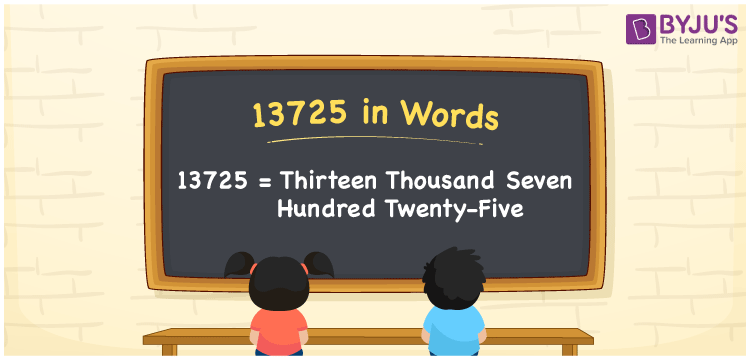# 13725 in Words

13725 in words is written as Thirteen thousand seven hundred twenty-five. In both the International System of Numerals and the Indian System of Numerals, 13725 is written as Thirteen thousand seven hundred twenty-five. The number 13725 is a Cardinal Number as it denotes some quantity. For example, “that mobile phone costs 13725 rupees”.

 13725 in Words Thirteen thousand seven hundred twenty-five Thirteen thousand seven hundred twenty-five in Number 13725

## 13725 in English Words

13725 in English words is read as “Thirteen thousand seven hundred twenty-five”.## How to Write 13725 in Words?

To write 13725 in words, we shall use the place value chart. In the place value chart, put 1 in the ten thousands, 3 in the thousands, 7 in the hundreds, 2 in the tens and 5 in the ones. Let us make a place value chart to write the number 13725 in words.

 Ten Thousands Thousands Hundreds Tens Ones 1 3 7 2 5

Thus, we can write the expanded form as

1 × Ten Thousand + 3 × Thousand + 7 × Hundred + 2 × Ten + 5 × One

= 1 × 10000 + 3 × 1000 + 7 × 100 + 2 × 10 + 5 × 1

= 10000 + 3000 + 700 + 20 + 5

= 13725

= Thirteen thousand seven hundred twenty-five.

13725 is a natural number, the successor of 13724 and the predecessor of 13726.

13725 in words – Thirteen thousand seven hundred twenty-five

• Is 13725 an odd number? – Yes
• Is 13725 an even number? – No
• Is 13725 a perfect square number? – No
• Is 13725 a perfect cube number? – No
• Is 13725 a prime number? – No
• Is 13725 a composite number? – Yes

## Frequently Asked Questions on 13725 in Words

Q1

### How to write 13725 in words?

13725 in words is written as Thirteen thousand seven hundred twenty-five.
Q2

### How to write 13725 in the International and Indian System of Numerals?

In both, the system of numerals, 13725 in words, is written as Thirteen thousand seven hundred twenty-five.
Q3

### How to write 13725 in a place value chart?

In the place value chart, write 1 in the ten thousands, 3 in the thousands, 7 in the hundreds, 2 in the tens and 5 in the ones, respectively.